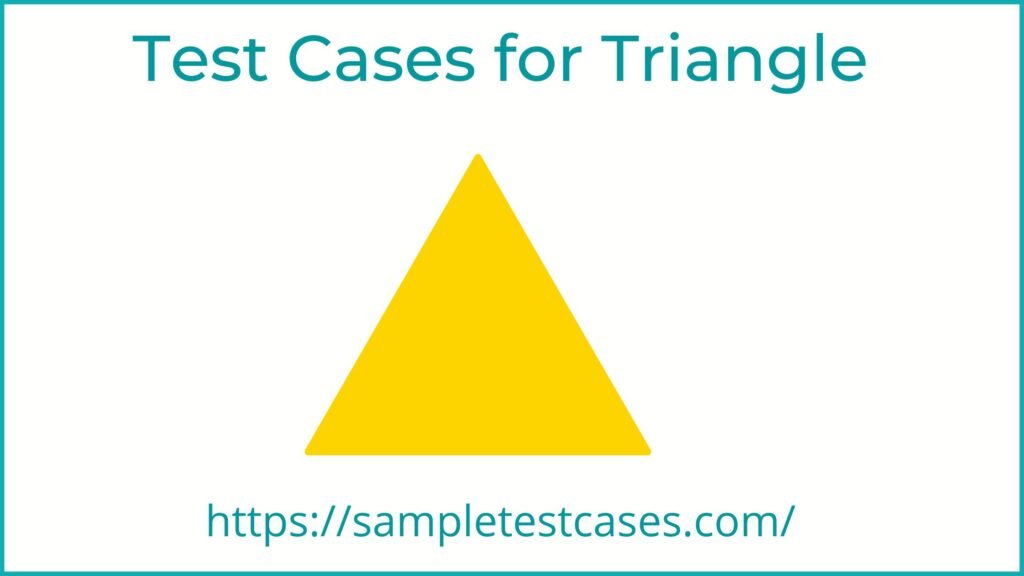# Test Cases for Triangle

Test Cases for Triangle Problem – Testing the properties of a triangle is a fundamental aspect of geometry and math education. A triangle is a three-sided polygon that can be classified according to its angles or side lengths. In this article, we will discuss the different test cases that can be used to classify a triangle and ensure that its properties are being accurately represented.

## Test Cases For Triangle

1. Verify that the triangle is two dimensional or not.
2. Verify that the triangle figure is closed(Polygon) or  not.
3. Verify that the triangle figure should be have three sides.
4. Verify that the triangle figure should be have three vertices.
5. Verify that the triangle figure should be have three angles.
6. Verify that the sum of the angles is 180 degree.
7. Verify that the triangle figure has minimum sides are three.
8. Verify that the triangle figure has maximum sides are three.
9. Verify that the triangle has three distinct and non-collinear points.
10. Verify that sum of the interior angles of the triangle is equal to 180 degrees.
11. Verify that the triangle is classified as acute, right, or obtuse based on its angle measures.
12. Verify that the triangle is classified as equilateral, isosceles, or scalene based on its side lengths.
13. Verify that the area of the triangle is correctly calculated using Heron’s formula or half the base times the height.
14. Verify that the parameter of the triangle is correctly calculated as the sum of the side lengths.
15. Verify that the triangle has special properties such as right triangle or isosceles triangle are correctly identified.
16. Verify that the triangle is placed in a 2D space and the coordinates of its vertices are provided and are used to calculate the area and perimeter.

## Negative Test Cases For triangle

1. Verify that the sum of any two sides is not greater than the third side (violation of triangle inequality theorem)
2. Verify that if there is not all three sides are provided
3. Verify that if any of the three sides is not a finite number (e.g. NaN, infinity)
4. Verify that any of the three sides is too large or too small to be a realistic side length of a triangle in the real world.

## Conclusion

In conclusion, testing the properties of a triangle is an essential aspect of understanding and working with this fundamental geometric shape. By using the appropriate test cases, we can classify a triangle according to its angles and side lengths, and ensure that its properties are being accurately represented. By thoroughly testing the properties of a triangle, we can deepen our understanding of this shape and improve our ability to work with it in mathematical and geometric contexts.

Scroll to Top